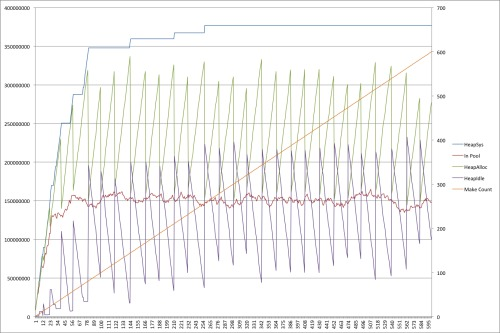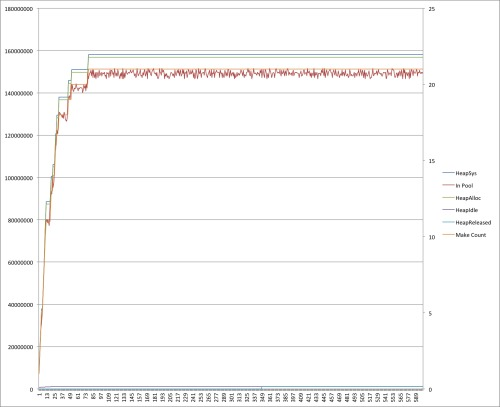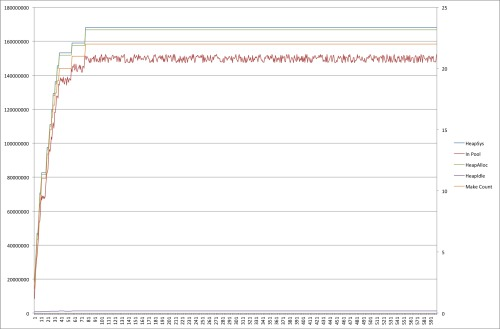# golang手动管理内存

2013/11/09 11:28

package main

import (
"fmt"
"math/rand"
"runtime"
"time"
)

func makeBuffer() []byte {
return make([]byte, rand.Intn(5000000)+5000000)
}

func main() {
pool := make([][]byte, 20)

var m runtime.MemStats
makes := 0
for {
b := makeBuffer()
makes += 1
i := rand.Intn(len(pool))
pool[i] = b

time.Sleep(time.Second)

bytes := 0

for i := 0; i < len(pool); i++ {
if pool[i] != nil {
bytes += len(pool[i])
}
}

fmt.Printf("%d,%d,%d,%d,%d,%d\n", m.HeapSys, bytes, m.HeapAlloc,
m.HeapIdle, m.HeapReleased, makes)
}
}

HeapSys：程序向应用程序申请的内存

HeapAlloc：堆上目前分配的内存

HeapIdle：堆上目前没有使用的内存

HeapReleased：回收到操作系统的内存

GC在Golang中运行的很频繁（参见GOGC环境变量（GOGC environment variable ）来理解怎样控制垃圾回收操作），因此在运行中由于一些内存被标记为”未使用“，堆上的内存大小会发生变化：这会导致HeapAlloc和HeapIdle发生变化。Golang中的scavenger 会释放那些超过5分钟仍然没有再使用的内存，因此HeapReleased不会经常变化。(在这张和后续的图中，左轴以是以byte为单位的内存大小，右轴是程序执行次数)

package main

import (
"fmt"
"math/rand"
"runtime"
"time"
)

func makeBuffer() []byte {
return make([]byte, rand.Intn(5000000)+5000000)
}

func main() {
pool := make([][]byte, 20)

buffer := make(chan []byte, 5)

var m runtime.MemStats
makes := 0
for {
var b []byte
select {
case b = <-buffer:
default:
makes += 1
b = makeBuffer()
}

i := rand.Intn(len(pool))
if pool[i] != nil {
select {
case buffer <- pool[i]:
pool[i] = nil
default:
}
}

pool[i] = b

time.Sleep(time.Second)

bytes := 0
for i := 0; i < len(pool); i++ {
if pool[i] != nil {
bytes += len(pool[i])
}
}

fmt.Printf("%d,%d,%d,%d,%d,%d\n", m.HeapSys, bytes, m.HeapAlloc,
m.HeapIdle, m.HeapReleased, makes)
}
}select {

case b = <- buffer:

default :

makes += 1

b = makeBuffer()

}

select {

case buffer <- pool[i]:

pool[i] = nil

default:

}

package main

import (
"container/list"
"fmt"
"math/rand"
"runtime"
"time"
)

var makes int
var frees int

func makeBuffer() []byte {
makes += 1
return make([]byte, rand.Intn(5000000)+5000000)
}

type queued struct {
when time.Time
slice []byte
}

func makeRecycler() (get, give chan []byte) {
get = make(chan []byte)
give = make(chan []byte)

go func() {
q := new(list.List)
for {
if q.Len() == 0 {
q.PushFront(queued{when: time.Now(), slice: makeBuffer()})
}

e := q.Front()

timeout := time.NewTimer(time.Minute)
select {
case b := <-give:
timeout.Stop()
q.PushFront(queued{when: time.Now(), slice: b})

case get <- e.Value.(queued).slice:
timeout.Stop()
q.Remove(e)

case <-timeout.C:
e := q.Front()
for e != nil {
n := e.Next()
if time.Since(e.Value.(queued).when) > time.Minute {
q.Remove(e)
e.Value = nil
}
e = n
}
}
}

}()

return
}

func main() {
pool := make([][]byte, 20)

get, give := makeRecycler()

var m runtime.MemStats
for {
b := <-get
i := rand.Intn(len(pool))
if pool[i] != nil {
give <- pool[i]
}

pool[i] = b

time.Sleep(time.Second)

bytes := 0
for i := 0; i < len(pool); i++ {
if pool[i] != nil {
bytes += len(pool[i])
}
}

fmt.Printf("%d,%d,%d,%d,%d,%d,%d\n", m.HeapSys, bytes, m.HeapAlloc
m.HeapIdle, m.HeapReleased, makes, frees)
}
}### 作者的其它热门文章

3
27 收藏

5 评论
27 收藏
3